Courses

# Test: Trigonometry- 2

## 15 Questions MCQ Test Quantitative Aptitude for GMAT | Test: Trigonometry- 2

Description
This mock test of Test: Trigonometry- 2 for GMAT helps you for every GMAT entrance exam. This contains 15 Multiple Choice Questions for GMAT Test: Trigonometry- 2 (mcq) to study with solutions a complete question bank. The solved questions answers in this Test: Trigonometry- 2 quiz give you a good mix of easy questions and tough questions. GMAT students definitely take this Test: Trigonometry- 2 exercise for a better result in the exam. You can find other Test: Trigonometry- 2 extra questions, long questions & short questions for GMAT on EduRev as well by searching above.
QUESTION: 1

### Two ships are sailing in the sea on the two sides of a lighthouse. The angle of elevation of the top of the lighthouse is observed from the ships are 30º and 45º respectively. If the lighthouse is 100 m high, the distance between the two ships is:​

Solution: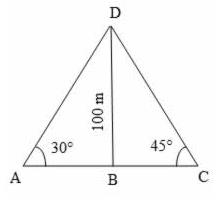Let BD be the lighthouse and A and C be the positions of the ships.
Then, BD = 100 m,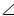BAD = 30° ,BCD = 45°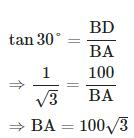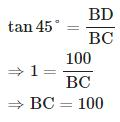Distance between the two ships
= AC = BA + BC
=100√3+100=100(√3+1)=1003+100=100(3+1)
= 100(1.73+1) = 100 × 2.73 = 273 m

QUESTION: 2

### A man standing at a point P is watching the top of a tower, which makes an angle of elevation of 30º with the man's eye. The man walks some distance towards the tower to watch its top and the angle of the elevation becomes 45º. What is the distance between the base of the tower and the point P?

Solution: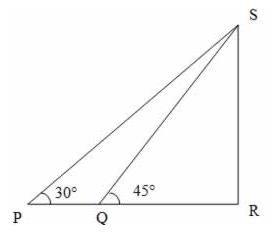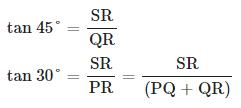Two equations and 3 variables. Hence we can not find the required value with the given data.

(Note that if one of SR, PQ, QR is known, this becomes two equations and two variables and if that was the case, we could have found out the required value.)

QUESTION: 3

### From a point P on a level ground, the angle of elevation of the top tower is 30º. If the tower is 200 m high, the distance of point P from the foot of the tower is:

Solution: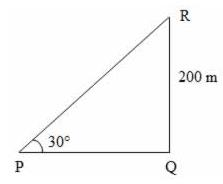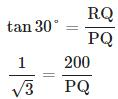PQ=200√3=200×1.73=346 m

QUESTION: 4

The angle of elevation of the sun, when the length of the shadow of a tree is equal to the height of the tree, is:

Solution:Consider the diagram shown above where QR represents the tree and PQ represents its shadow
We have, QR = PQ
Let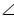QPR = θ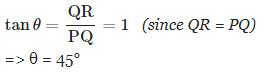i.e., required angle of elevation = 45°

QUESTION: 5

An observer 2 m tall is 10√3 m away from a tower. The angle of elevation from his eye to the top of the tower is 30º. The height of the tower is:

Solution: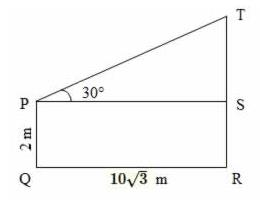SR = PQ = 2 m
PS = QR =10√3m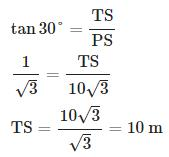TR = TS + SR = 10 + 2 = 12 m

QUESTION: 6

The angle of elevation of a ladder leaning against a wall is 60º and the foot of the ladder is 12.4 m away from the wall. The length of the ladder is:

Solution: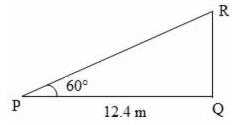Consider the diagram shown above where PR represents the ladder and RQ represents the wall.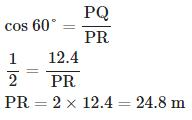QUESTION: 7

A man on the top of a vertical observation tower observers a car moving at a uniform speed coming directly towards it. If it takes 8 minutes for the angle of depression to change from 30° to 45°, how soon after this will the car reach the observation tower?

Solution: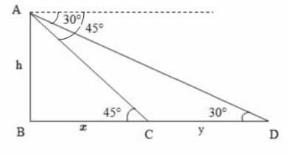Consider the diagram shown above. Let AB be the tower. Let D and C be the positions of the car.

Then, ∠ADC = 30° , ∠ACB = 45°

Let AB = h, BC = x, CD = y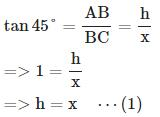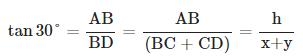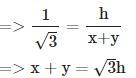⇒ y = √3h−h  (∵ Substituted the value of x from equation 1 )
⇒y = h(√3−1)
Given that distance y is covered in 8 minutes.
i.e, distance h(√3−1) is covered in 8 minutes.
Time to travel distance x
= Time to travel distance h (∵ Since x = h as per equation 1).
Let distance h is covered in t minutes.
since distance is proportional to the time when the speed is constant, we have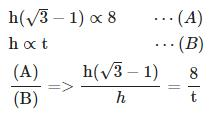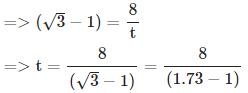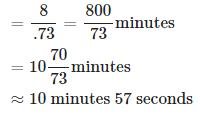QUESTION: 8

A man is watching from the top of a tower a boat speeding away from the tower. The boat makes an angle of depression of 45° with the man's eye when at a distance of 100 metres from the tower. After 10 seconds, the angle of depression becomes 30°. What is the approximate speed of the boat, assuming that it is running in still water?

Solution: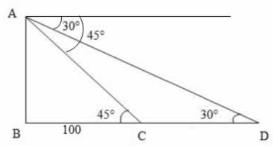Consider the diagram shown above.
Let AB be the tower. Let C and D be the positions of the boat
Then,ACB = 45° ,ADC = 30°, BC = 100 m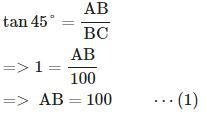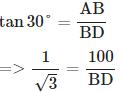(∵ Substituted the value of AB from equation 1)

=> BD =100√3

CD = (BD - BC) =(100√3−100)=100(√3−1)
It is given that the distance CD is covered in 10 seconds.
i.e., the distance 100(√3−1) is covered in 10 seconds.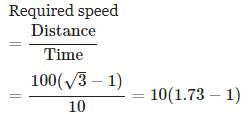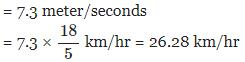QUESTION: 9

The top of a 15 metre high tower makes an angle of elevation of 60° with the bottom of an electronic pole and angle of elevation of 30° with the top of the pole. What is the height of the electric pole?

Solution: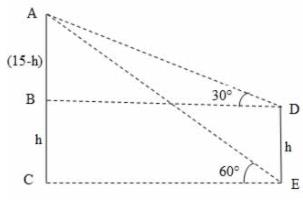Consider the diagram shown above. AC represents the tower and DE represents the pole.
Given that AC = 15 m,ADB = 30°,AEC = 60°
Let DE = h
Then, BC = DE = h,
AB = (15-h)    (∵ AC=15 and BC = h),
BD = CE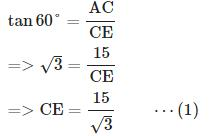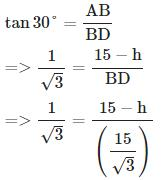(∵ BD = CE and substituted the value of CE from equation 1 )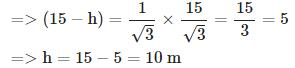i.e., height of the electric pole = 10 m

QUESTION: 10

The angle of elevation of the top of a tower from a certain point is 30°. If the observer moves 40 m towards the tower, the angle of elevation of the top of the tower increases by 15°. The height of the tower is:

Solution: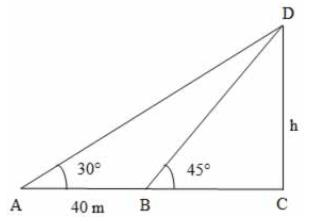Let DC be the tower and A and B be the positions of the observer such that AB = 40 m
We haveDAC = 30°,DBC = 45°
Let DC = h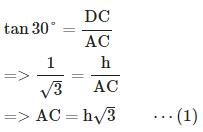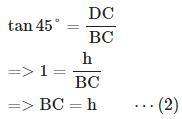We know that, AB = (AC - BC)
=> 40 = (AC - BC)
=> 40=(h√3−h)40= [∵ from (1) & (2)]
=>40=h(√3−1)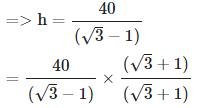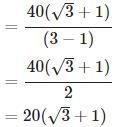=20(1.73+1)=20×2.73=54.6 m

QUESTION: 11

On the same side of a tower, two objects are located. Observed from the top of the tower, their angles of depression are 45° and 60°. If the height of the tower is 600 m, the distance between the objects is approximately equal to :

Solution: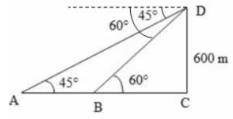Let DC be the tower and A and B be the objects as shown above.
Given that DC = 600 m ,DAC = 45°,DBC = 60°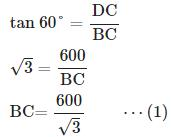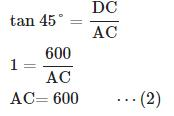Distance between the objects
= AB = (AC - BC)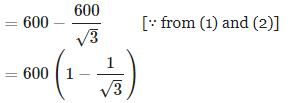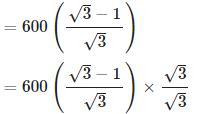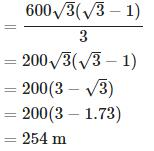QUESTION: 12

A ladder 10 m long just reaches the top of a wall and makes an angle of 60° with the wall.Find the distance of the foot of the ladder from the wall (√3=1.73)

Solution: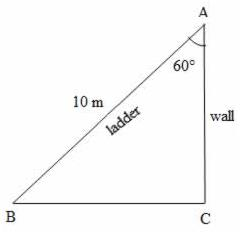Let BA be the ladder and AC be the wall as shown above.
Then the distance of the foot of the ladder from the wall = BC
Given that BA = 10 m ,BAC = 60°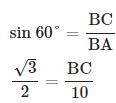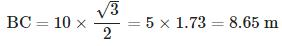QUESTION: 13

From a tower of 80 m high, the angle of depression of a bus is 30°. How far is the bus from the tower?

Solution: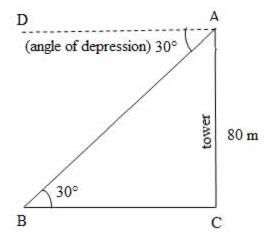Let AC be the tower and B be the position of the bus.
Then BC = the distance of the bus from the foot of the tower.
Given that height of the tower, AC = 80 m and the angle of depression,DAB = 30°ABC =DAB = 30°   (because DA || BC)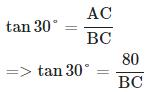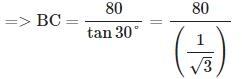=80×1.73=138.4 mtan⁡30°=ACBC=>tan⁡30°=80BC=>BC = 80tan⁡30°=80(13)=80×1.73=138.4 m
i.e., Distance of the bus from the foot of the tower = 138.4 m

QUESTION: 14

The angle of elevation of the top of a lighthouse 60 m high, from two points on the ground on its opposite sides are 45° and 60°. What is the distance between these two points?

Solution: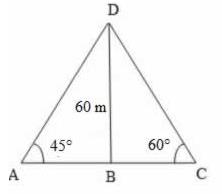Let BD be the lighthouse and A and C be the two points on ground.
Then, BD, the height of the lighthouse = 60 mBAD = 45° ,BCD = 60°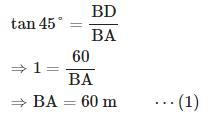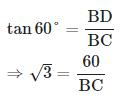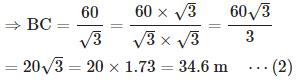Distance between the two points A and C
= AC = BA + BC
= 60 + 34.6   [∵ Substituted value of BA and BC from (1) and (2)]
= 94.6 m

QUESTION: 15

From the top of a hill 100 m high, the angles of depression of the top and bottom of a pole are 30° and 60° respectively. What is the height of the pole?

Solution: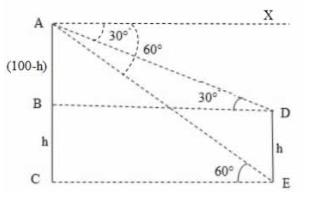Consider the diagram shown above. AC represents the hill and DE represents the pole

Given that AC = 100 m
∠XAE = ∠AEC = 60°   (∵ AX || CE)
Let DE = h
Then, BC = DE = h,
AB = (100-h)   (∵ AC=100 and BC = h),
BD = CE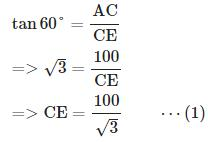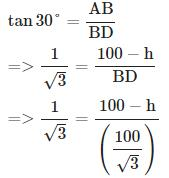(∵ BD = CE and substituted the value of CE from equation 1)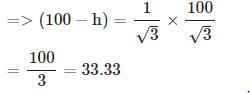⇒ h=100−33.33=66.67 m
i.e., the height of the pole = 66.67 m